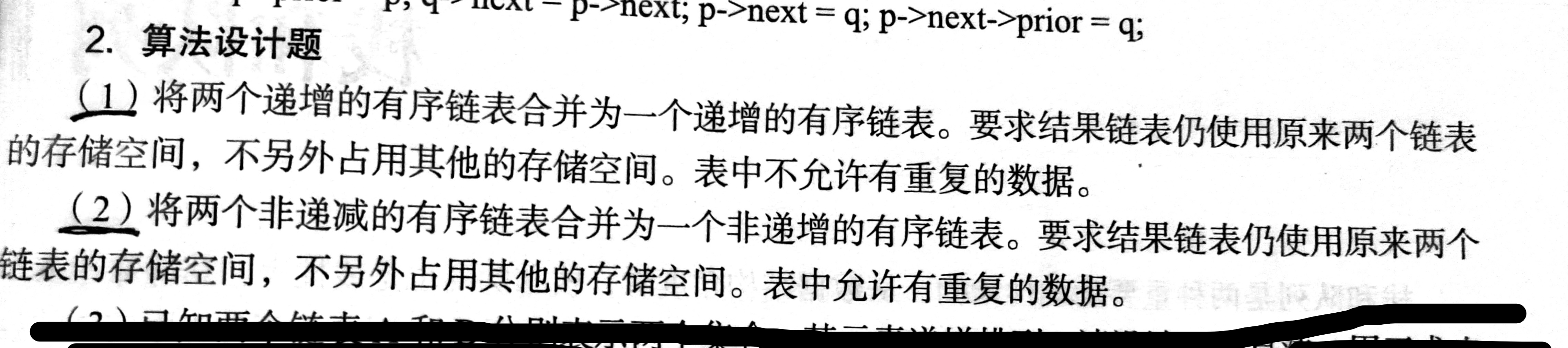• } //将两个递增有序链表合并为一个递增的有序链表 public void mergeSurgeList(LinkList La) { if (La==null) { System.out.println("Error"); return ; } if(!isSurge(La)) { System.out.println("No surge"); ...代码如下:

package sjjgniub;

import java.util.Scanner;

@SuppressWarnings("all")

private class Node
{
int data;
Node next;

public Node()
{

}

public Node(int data)
{
this.data = data;
next = null;
}
}

{
System.out.println("Error");
return;
}
}

public void createList(int[] arr)
{
for (int i = 0;i<arr.length;i++) {
Node s = new Node(arr[i]);
p.next = s;
p = s;
}
}

public void createList()
{
Scanner sc = new Scanner(System.in);
int n = sc.nextInt();
for (int i = 0;i<n;i++)
{
int temp = sc.nextInt();
Node s = new Node(temp);
p.next = s;
p = s;

}
}

public void printList()
{
while(p!=null)
{
System.out.print(p.data+" ");
p = p.next;
}
System.out.println();
}

//判断是否为递增有序链表
{

if (L==null)
{
return false;
}
{
return true;
}
while(p.next!=null)
{
if (p.next.data < p.data)
{
return false;
}
p = p.next;
}
return true;
}

//将两个递增的有序链表合并为一个递增的有序链表
{
if (La==null)
{
System.out.println("Error");
return ;
}

if(!isSurge(La))
{
System.out.println("No surge");
return ;
}

if (!isSurge(this))
{
System.out.println("No surge");
return ;
}

while(p1!=null && p2!=null)
{
if (p1.data > p2.data)
{
pc.next = p2;
p2 = p2.next;
pc = pc.next;
}
else if (p1.data < p2.data)
{
pc.next = p1;
p1 = p1.next;
pc = pc.next;
}
else
{
pc.next = p1;
p1 = p1.next;
p2 = p2.next;
pc = pc.next;
}
}
if (p1==null && p2==null)
{
pc.next = null;
}
else if (p1==null)
{
pc.next = p2;

}
else if (p2==null)
{
pc.next = p1;
}
La = null;

}

//将两个非递减的有序链表合并为一个非递增的有序链表
{
if (La==null)
{
System.out.println("Error");
return ;
}
if(!isSurge(La))
{
System.out.println("No surge");
return ;
}

if (!isSurge(this))
{
System.out.println("No surge");
return ;
}

pc.next = null;
while(p1!=null&& p2!=null)
{
if (p1.data >= p2.data)
{
Node tmp = p2.next;
p2.next = pc.next;
pc.next = p2;
p2 = tmp;
}
else
{
Node tmp = p1.next;
p1.next = pc.next;
pc.next = p1;
p1 = tmp;
}
}

while(p2!=null)
{
Node tmp = p2.next;
p2.next = pc.next;
pc.next = p2;
p2 = tmp;
}

while(p1!=null)
{
Node tmp = p1.next;
p1.next = pc.next;
pc.next = p1;
p1 = tmp;
}

La = null;

}

}

测试类：

package sjjgniub;

public class TestList {
public static void main(String[] args) {
int[] arr1 = {1,56,234,423,1000};
int[] arr2 = {213,423,1000};
System.out.println();

}
}

展开全文链表 java 数据结构
• 题目：假设有两个按元素值依次递增次序排列的线性表，均以单链表的形式存储。请编写算法两个单链表归并为一个按元素值递减次序排列的单链表，并要求利用原来两个单链表的结点存放归并后的单链表。 分析：意思...

题目假设有两个按元素值依次递增次序排列的线性表，均以单链表的形式存储。请编写算法将这两个单链表归并为一个按元素值递减次序排列的单链表，并要求利用原来两个单链表的结点存放归并后的单链表。

分析：意思就是两条递增链表合并成为一条递减链表，然后还不能重新创建新的链表，要在原有的两条链表上修改，要最终的链表逆序，所以使用头插法，将AB两条链表的元素进行比较，较小的进行头插，和Day2类似，Day2时顺序表，这个是链表，大同小异。

算法思想：A、B分别设置指针p、q，依次比较p->data和q->data，然后将较小的结点头插进合并链表中，直到遍历完两条链表，遍历过程中如果其中一条链表为空了，那么就将剩下的链表依次头插进合并链表即可。

代码

LNode *p=A->next; // 结点初始化
LNode *q=B->next;
A->next=Null; // AB的头指针置空
B->next=Null;
while(p!=NULL && q!=NULL){
if(p->data<q->data){
r=p->next; // ❤头插法，将较小的进行头插
p->next=A->next;
A->next=p;
p=r;
}
else{
r=q->next;
q->next=A->next;
A->next=q;
q=r;
}
}
while(q!=NULL){ // 长度相等的部分遍历完了，B有剩余，将B剩下的元素全部依次进行头插即可
r=q->next;
q->next=A->next;
A->next=q;
q=r;
}
while(p!=NULL){ // 长度相等的部分遍历完了，A有剩余，将A剩下的元素全部依次进行头插即可
r=p->next;
p->next=A->next;
A->next=p;
p=r;
}
}

展开全文链表 数据结构
• 代码注释处的两个排序函数有BUG，先放着，等以后看看错在哪里。 第三个排序函数没问题，可正常运行。 #include<iostream> using namespace std; typedef struct LNode { int data; struct LNode* next; int...

可正常运行。

#include<iostream>
using namespace std;
typedef struct LNode
{
int data;
struct LNode* next;
int num;

{
L = new LNode;
L->next = NULL;
L->num = 0;
for (int i = 0; i < n; i++)
{
LNode* p = new LNode;
cin >> p->data;
p->next = NULL;
r->next = p;
r = p;
L->num++;
}
return L;
}

{
if (L->num == 0)
cout << "链表为空！" << endl;
else
{
int q = L->num;
for (int i = 0; i < q; i++)
{
if (L->next->data != NULL)
{
cout << L->next->data << " ";
L = L->next;
}
}
cout << endl;
}
}

{
L3 = L1;
while (pa && pb)
{
if (pa->data <= pb->data)
{
pc->next = pa;
pc = pa;
pa = pa->next;
}
else
{
pc->next = pb;
pc = pb;
pb = pb->next;
}
}
pc->next = pa ? pa : pb;
L3->num = L1->num + L2->num;
return L3;
}

{
while (p != NULL)
{
while (q != NULL)
{
if (p->data < q->data)
{
int temp = p->data;
p->data = q->data;
q->data = temp;

}
q = q->next;
}
p = p->next;
}
return L;
}

int main()
{
int n1, n2;
cout << "请输入第一个非递减链表的长度：" << endl;
cin >> n1;
cout << "请输入第一个非递减链表的内容：" << endl;
L1 = createList(L1, n1);
cout << "请输入第二个非递减链表的长度：" << endl;
cin >> n2;
cout << "请输入第二个非递减链表的内容：" << endl;
L2 = createList(L2, n2);
cout << "第一个链表为：" << endl;
showList(L1);
cout << "第二个链表为：" << endl;
showList(L2);
sortList(L3);
cout << "合并后非递增链表为：" << endl;
showList(L3);
return 0;

}

展开全文数据结构
• 问题：将两个递增有序链表合并为一个递增的有序链表。要求结果链表仍使用原来两个链表的存储空间, 不另外占用其它的存储空间。表中不允许有重复的数据。 分析： 合并后的新表使用头指针Lc指向，pa和pb分别是链表La...

问题：将两个递增的有序链表合并为一个递增的有序链表。要求结果链表仍使用原来两个链表的存储空间, 不另外占用其它的存储空间。表中不允许有重复的数据。

分析：
合并后的新表使用头指针Lc指向，pa和pb分别是链表La和Lb的工作指针,初始化为相应链表的第一个结点，从第一个结点开始进行比较，当两个链表La和Lb均为到达表尾结点时，依次摘取其中较小者重新链接在Lc表的最后。如果两个表中的元素相等，只摘取La表中的元素，删除Lb表中的元素，这样确保合并后表中无重复的元素。当一个表到达表尾结点，为空时，将非空表的剩余元素直接链接在Lc表的最后。

pa=La->next;
pb=Lb->next;//pa和pb分别是链表La和Lb的工作指针,初始化为相应链表的第一个结点
Lc=pc=La;//用La的头结点作为Lc的头结点
while(pa && pb) {
if(pa->data < pb->data) {
//取较小者La中的元素，将pa链接在pc的后面，pa指针后移
pc->next=pa;
pc=pa;
pa=pa->next;
}
else if(pa->data > pb->data) {
//取较小者Lb中的元素，将pb链接在pc的后面，pb指针后移
pc->next=pb;
pc=pb;
pb=pb->next;
}
else {
//相等时取La中的元素，删除Lb中的元素
pc->next=pa;
pc=pa;
pa=pa->next;
q=pb->next;
delete pb;
pb=q;
}
}
pc->next=pa?pa:pb;//插入剩余段
delete Lb; //释放Lb的头结点
}

展开全文链表 数据结构
• 将两个递增有序链表合并为一个非递减的有序链表 将两个递增有序链表合并为一个非递减的有序链表。要求结果链表仍使用原来两个链表的存储空间, 不另外占用其它的存储空间。表中允许有重复的数据。操作的名称...
• 将两个递增有序链表合并为一个递增的有序链表。要求结果链表仍使用原来两个链表的存储空间, 不另外占用其它的存储空间。表中不允许有重复的数据。 #include<stdio.h> #include<malloc.h> typedef ...链表 数据结构 c语言 算法链表 数据结构
• 将两个递增有序链表合并为一个非递减的有序链表。要求结果链表仍使用原来两个链表的存储空间, 不另外占用其它的存储空间。表中允许有重复的数据操作的名称和参数使用下列结构：La和Lb表示将要合并的链表，合并后...
• //p插入到链表的尾部 r=p;//指针r又指向链表尾部 p2=p2->next;//L2里的指针向后移动一步 } else { p=new LNode; p->data=p1->data; p->next=NULL; r->next=p; r=p; p1=p1->next; } } if(p1==NULL)//表1结束了，放...
• 输入两个单调递增链表，输出两个链表合成后的链表，当然我们需要合成后的链表满足单调不减规则。 示例1 输入：{1,3,5},{2,4,6} 返回：{1,2,3,4,5,6}解题思路 定义两个指针，指向一条带头节点的空链表 一个用作...数据结构 链表
• 将两个非递减的有序链表合并为一个非递增有序链表。要求结果链表仍使用原来两个链表的存储空间, 不另外占用其它的存储空间。表中允许有重复的数据 由于最近数据结构课布置了这个作业，并且知识一知半解（练习少了...数据结构 链表 指针
• //将两个非递减链表合并为一个非递增有序链表，只用La,Lb已有的存储空间。 LinkList pa,pb; pa=La->next; //pa,pb用来存储La,Lb的首元结点的地址 pb=Lb->next; free(Lb); //释放Lb的头结点 La->next=NULL; //令...
• //将两个非递减的有序链表合并为一个非递增有序链表 #include<iostream> #include<stdlib.h> using namespace std; typedef struct Lnode { //定义链表 int data; struct Lnode* next; }link; ...链表 c++ 数据结构
•  写一个函数SortedMerge函数，该函数有两个参数，都是递增的链表，函数的功能就是合并这两个递增链表为一个递增的链表，SortedMerge的返回值是新的链表。新链表由前两个链表按元素递增顺序合并而成，也就是说它...面试题 指针 算法
• 将两个有序链表合并为一个新链表，要求新的链表是通过拼接两个链表的节点来生成的，且合并后新链表依然有序。 比如{1，4} {3，5}，合并就是{1，3，4，5}； 解题思路 首先我们要判定是否有一个链表为空，都为空，...
• 思路：可以利用这两个链表本来就是递增有序的特性进行合并，然后再进行逆序，即可得到递减有序链表。 链表类： //链表类 class List { public: int val; List* next; public: List() : val(0), next(nullptr) {}...链表 数据结构 list
• 分享给大家供大家参考，具体如下：将两个有序链表合并为一个新的有序链表并返回。新链表是通过拼接给定的两个链表的所有节点组成的。示例：输入：1->2->4, 1->3->4输出：1->1->2->3->4->...
• 1. 合并两个有序链表 描述： 将两个升序链表合并为一个新的升序链表并返回。新链表是通过拼接给定的两个链表的所有节点组成的。 思路： （1）先定义一个傀儡指针ListNode perHead = new ListNode(-1)，再定义...java 算法 数据结构 链表
• 描述 给定两个递增的整数序列A和B，利用链表表示序列A和B，A和B合并为一个递增的有序序列C，序列C不允许有重复的数据。要求空间复杂度为O(1)。 输入 多组数据，每组数据有三行，第一行为序列A和B的长度n和m，第二...链表 数据结构 c++
• 合并两个有序链表题目描述：将两个有序链表合并为一个新的有序链表并返回。新链表是通过拼接给定的两个链表的所有节点组成的。示例：输入：1->2->4, 1->3->4输出：1->1->2->3->4->4解题...
• List Merge( List L1, List L2 ); 其中List结构定义如下： `c typedef struct Node *PtrToNode; struct Node { ...L1和L2是给定的带头结点的单链表，其结点存储的数据是递增有序的；函数Merge要数据结构 链表
• 本题要求实现一个函数，将两个链表表示的递增整数序列合并为一个非递减的整数序列。 //函数接口定义： List Merge( List L1, List L2 ); //其中List结构定义如下： typedef struct Node *PtrToNode; struct Node { ...
• 已知两个非降序链表序列S1与S2，设计函数构造出S1与S2合并后的新的非降序链表S3。输入格式:输入分两行，分别在每行给出由若干个正整数构成的非降序序列，用−1表示序列的结尾(−1不属于这个序列)。数字用空格间隔。...
• 将两个递增的有序顺序表合并成一个递增的有序链表，要求结果链表使用原来的存储空间，不占用额外的存储空间，表中不允许有其他重复的数据。 解答 假定创建链表输入的数据有序。 为了保证新表和原来一样有序，可以...
• 将两个升序链表合并为一个新的 升序 链表并返回。 新链表是通过拼接给定的两个链表的所有节点组成的。 例如： 输入：1->2->4, 1->3->4 输出：1->1->2->3->4->4 问题解析 先判断两个...java
• 输入两个递增排序的链表，合并这两个链表并使新链表中的节点仍然是递增排序的。 示例1： 输入：1->2->4, 1->3->4 输出：1->1->2->3->4->4 代码 struct ListNode* mergeTwoLists(struct ...
• 题目要求实现一个函数，将两个链表表示的递增整数序列合并为一个非递减的整数序列。这道题较为基础，主要考察C语言中链表的基本操作。只要会“连接”链表，考虑清楚比较过程和前后关系不难想出思路，关键是对链表的......